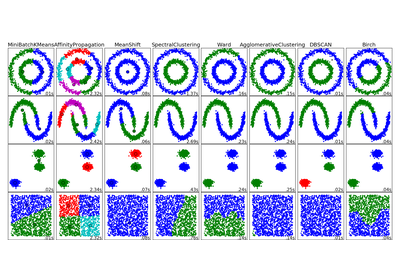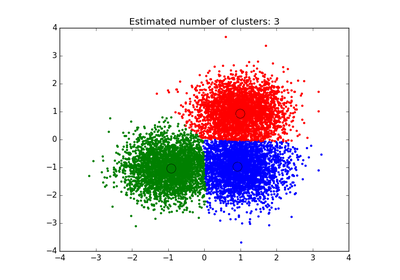# `sklearn.cluster`.estimate_bandwidth¶

`sklearn.cluster.``estimate_bandwidth`(X, quantile=0.3, n_samples=None, random_state=0, n_jobs=1)[source]

Estimate the bandwidth to use with the mean-shift algorithm.

That this function takes time at least quadratic in n_samples. For large datasets, it’s wise to set that parameter to a small value.

Parameters: X : array-like, shape=[n_samples, n_features] Input points. quantile : float, default 0.3 should be between [0, 1] 0.5 means that the median of all pairwise distances is used. n_samples : int, optional The number of samples to use. If not given, all samples are used. random_state : int or RandomState Pseudo-random number generator state used for random sampling. n_jobs : int, optional (default = 1) The number of parallel jobs to run for neighbors search. If `-1`, then the number of jobs is set to the number of CPU cores. bandwidth : float The bandwidth parameter.

## Examples using `sklearn.cluster.estimate_bandwidth`¶Comparing different clustering algorithms on toy datasetsA demo of the mean-shift clustering algorithm